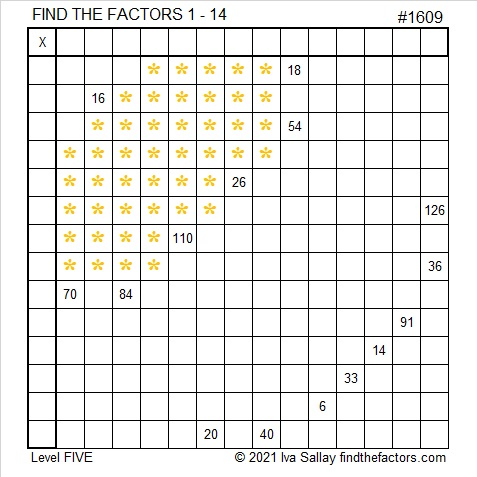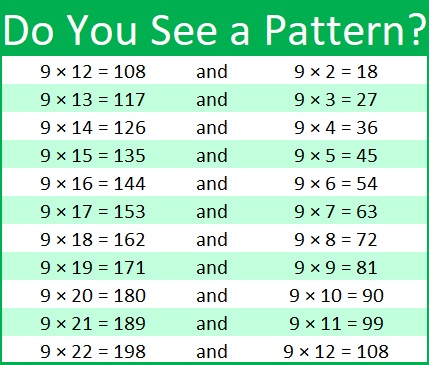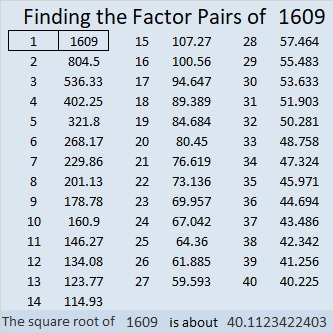# 1609 Pot of Gold

Contents

### Today’s Puzzle:

They say at the end of the rainbow, there is a pot of gold that belongs to some leprechaun. Because this is a Find the Factors 1 to 14 puzzle, this pot of gold has some choice mathematical nuggets. For example, is 7 or 14 the common factor of 70 and 84? Don’t guess which one is the common factor for the puzzle. Use logic to eliminate one of those possibilities instead.

Likewise, both 6 and 9 are common factors of 18 and 54. And 4, 5, and 10 are all common factors of 20 and 40. Logic will narrow each possibility down to one possible factor!Print the puzzles or type the solution in this excel file: 14 Factors 1604-1612.

I like that you also need to find the common factor of 126 and 36. I noticed a pattern with those clues. The pattern is limited to the multiplication facts given below, but I think it is still a pretty cool pattern.Here’s the pattern I saw for 126 and 36:

• Since 9 is one of the factors, the sum of the digits of any of the products equals 9.
• 1 + 2 = 3. The sum of the first two numbers of the product in the first column equals the first part of the product in the second column.
• Obviously, both clues end in 6 so the last digit of their other factors will end with the same number, 4.

That should give you a good start in solving the puzzle!

### Factors of 1609:

• 1609 is a prime number.
• Prime factorization: 1609 is prime.
• 1609 has no exponents greater than 1 in its prime factorization, so √1609 cannot be simplified.
• The exponent in the prime factorization is 1. Adding one to that exponent we get (1 + 1) = 2. Therefore 1609 has exactly 2 factors.
• The factors of 1609 are outlined with their factor pair partners in the graphic below.

How do we know that 1609 is a prime number? If 1609 were not a prime number, then it would be divisible by at least one prime number less than or equal to √1609. Since 1609 cannot be divided evenly by 2, 3, 5, 7, 11, 13, 17, 19, 23, 29, 31, or 37, we know that 1609 is a prime number.### More about the Number 1609:

1609² = 2588881. That’s a perfect square, followed by four 8’s, followed by a perfect square. OEIS.org reports that 1609² is the smallest perfect square with four 8’s in a row.

1609 is the sum of two squares:
40² + 3² = 1609.

1609 is the hypotenuse of a Pythagorean triple:
240-1591-1609, calculated from 2(40)(3), 40² – 3², 40² + 3².

Here’s another way we know that 1609 is a prime number: Since its last two digits divided by 4 leave a remainder of 1, and 40² + 3² = 1609 with 40 and 3 having no common prime factors, 1609 will be prime unless it is divisible by a prime number Pythagorean triple hypotenuse less than or equal to √1609. Since 1609 is not divisible by 5, 13, 17, 29, or 37, we know that 1609 is a prime number.

This site uses Akismet to reduce spam. Learn how your comment data is processed.